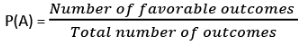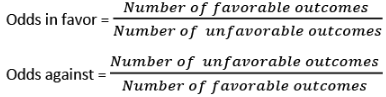Courses

# Important Formulae: Probability CAT Notes | EduRev

## Quant : Important Formulae: Probability CAT Notes | EduRev

The document Important Formulae: Probability CAT Notes | EduRev is a part of Quant category.
All you need of Quant at this link: Quant

Probability:For Complementary Events: P(A) + P(A’) = 1
For Exhaustive Events: P(A) + P(B) +P(C)… = 1

P (A U B) = P(A) + P(B) – P(A ∩ B)
For Mutually Exclusive Events P(A ∩ B) = 0
⇒ P (A U B) = P(A) + P(B)

Multiplication Rule:
P(A ∩ B) = P(A) P(B/A) = P(B) P(A/B)
For Independent Events P(A/B) = P(B) and P(B/A) = P(B)
⇒ P(A ∩ B) = P(A).P(B)
⇒ P (A U B) = P(A) + P(B) – P(A).P(B)

EduRev's Tip: If the probability of an event occurring is P, then the probability of that event occurring ‘r’ times in ‘n’ trials is = nCr x Pr x (1-P)n-r

OddsOffer running on EduRev: Apply code STAYHOME200 to get INR 200 off on our premium plan EduRev Infinity!

,

,

,

,

,

,

,

,

,

,

,

,

,

,

,

,

,

,

,

,

,

;